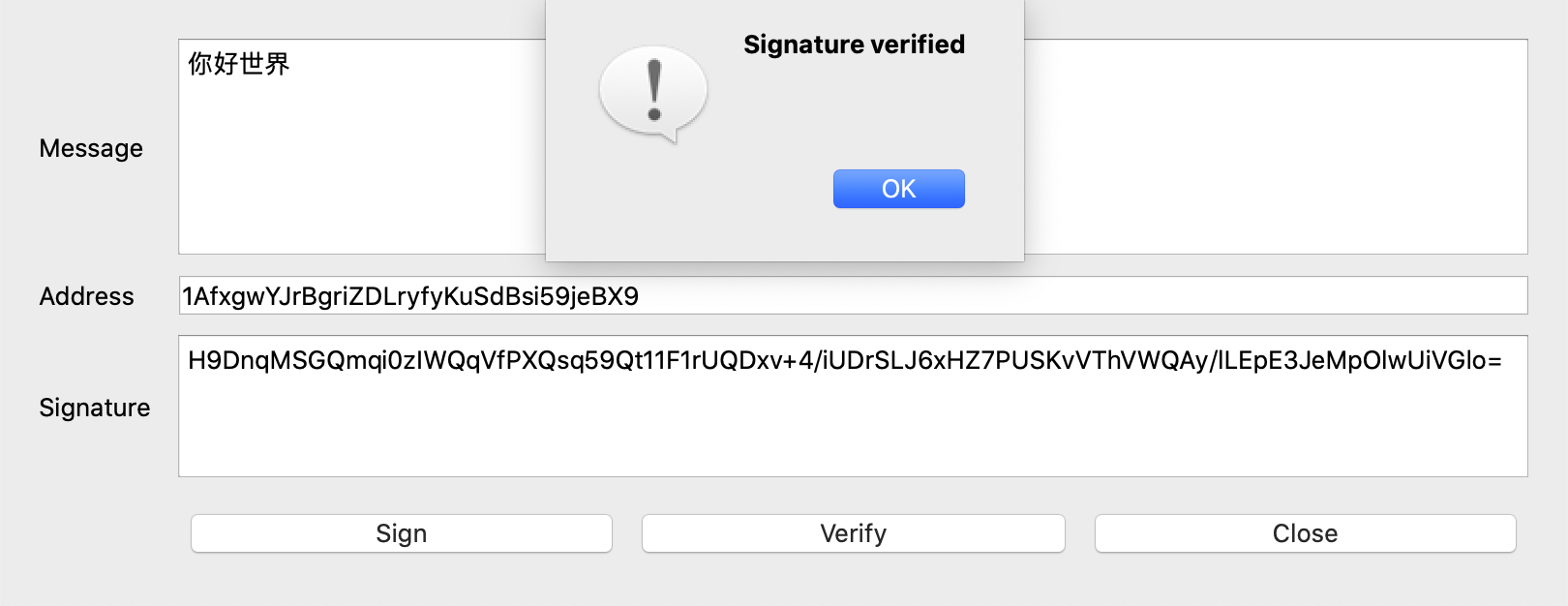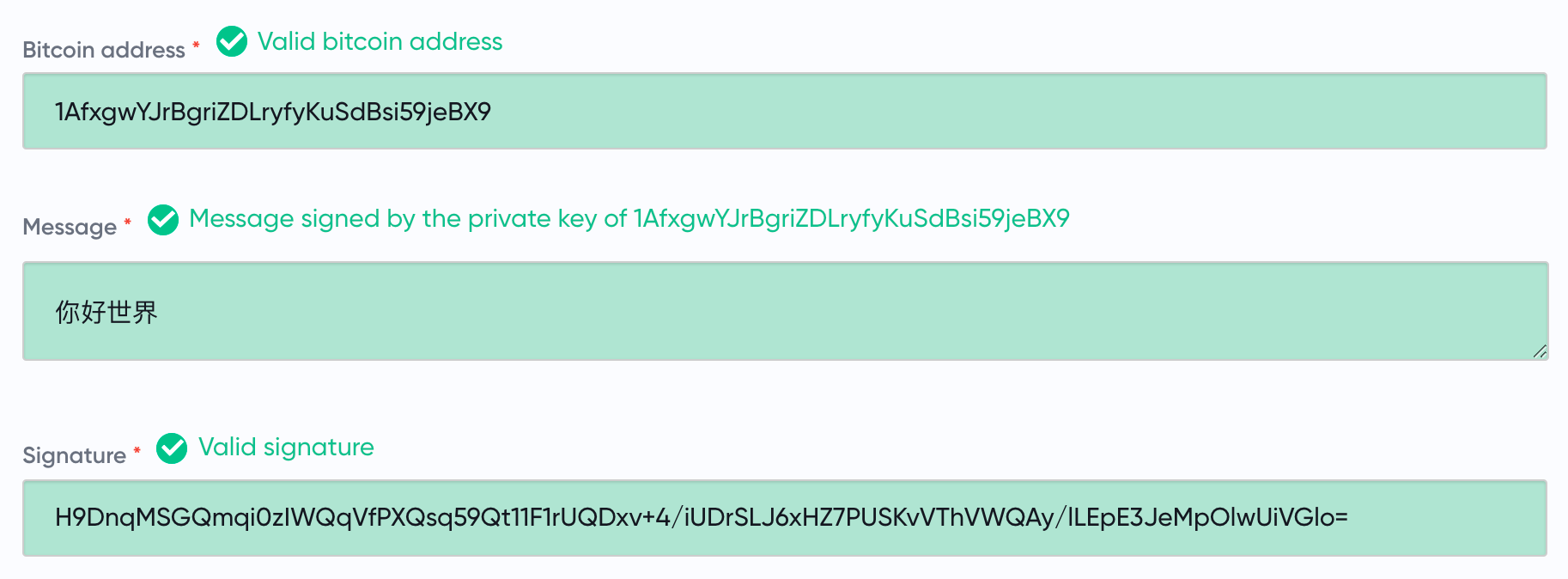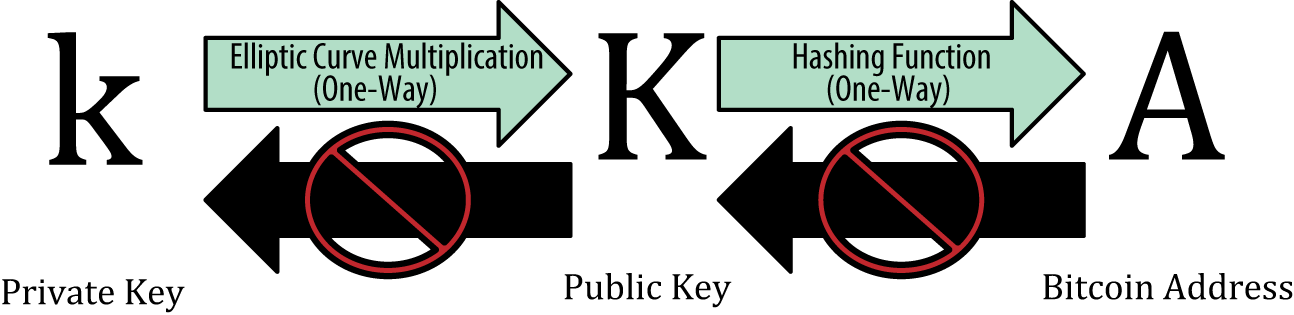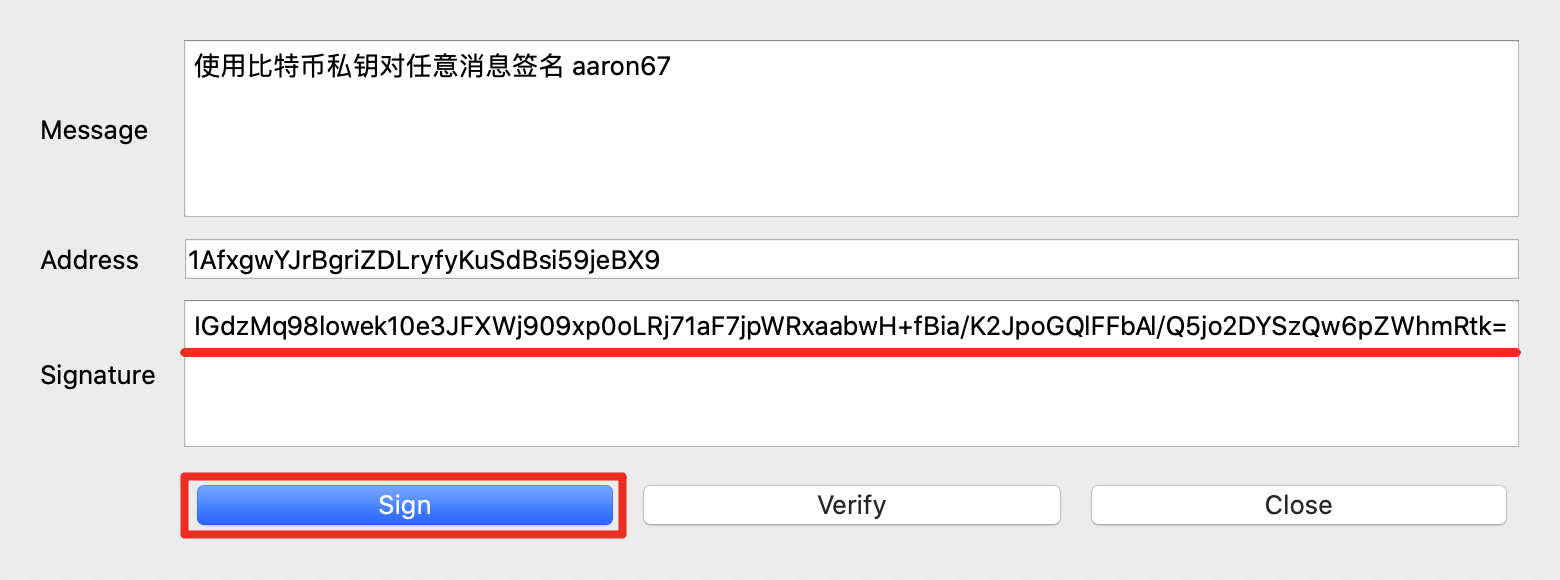• 对签名方，能提供正确的签名，就意味着他一定有私钥
• 对验证方，验签通过即可保证，消息内容未经篡改，且消息来源可靠# 发现“问题”# 再说 ECDSA 签名

$$a = r^{-1}(sk - e) \bmod{n}$$

$$A = aG = r^{-1}(sk - e) \cdot G = r^{-1}(s \cdot kG - eG) = r^{-1}(sK - eG)$$

• $x_K$ 与 $n$ 的大小关系。若 $x_K < n$ 则 $x_K = r$，反之 $x_K = r + n$
• $y_K$ 的奇偶性

# Recoverable ECDSA 签名

00 $x_K < n$，$y_K$ 是偶数 0
01 $x_K < n$，$y_K$ 是奇数 1
10 $x_K > n$，$y_K$ 是偶数 2
11 $x_K > n$，$y_K$ 是奇数 3

# 计算消息摘要

len(H) H 的字节长度，VarInt 类型表示，定值 0x18
H 字符串 Bitcoin Signed Message:\n 的 UTF-8 编码
len(M) M 的字节长度，VarInt 类型表示
M 消息 $m$ 的 UTF-8 编码

# 序列化签名结果

1 前缀信息
32 整数 r 按大端模式序列化后的字节流
32 整数 s 按大端模式序列化后的字节流

27 + recid + 4 公钥用 33 字节表示
27 + recid + 0 公钥用 65 字节表示# 验签

1. 从序列化后的签名中，解析出recid和 $(r, s)$，同时明确公钥的表示方式
2. 恢复公钥
3. 用公钥和消息验证签名 $(r, s)$
4. 验证恢复出的公钥的哈希，是否与输入的比特币地址相匹配

$$y^2 \equiv x^3 + 7 \pmod{p}$$

$$a^p \equiv a \pmod{p}$$

$$y^{p} \equiv y \pmod{p}$$

$$y^{p+1} \equiv y^2 \pmod{p}$$

$$Y^{\frac{p+1}{2}} \equiv Y \pmod{p}$$

$$y = Y^{\frac{p+1}{4}} \bmod{p}\ \ \ \ 或\ \ \ \ -Y^{\frac{p+1}{4}} \bmod{p}$$sign_message.py